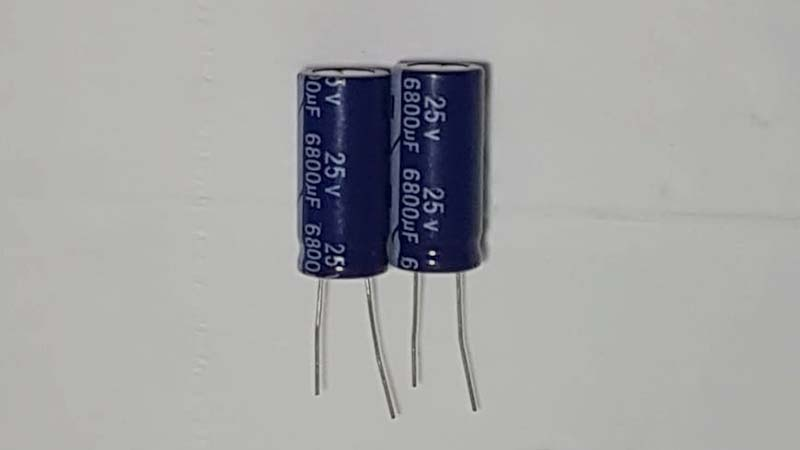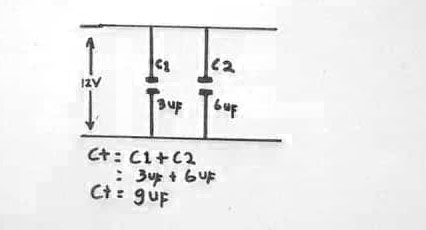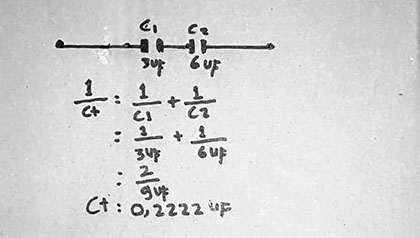# The Difference between Capacitors in Series Vs. Capacitors in Parallel

Capacitors with numerous connections can perform many different tasks. If a group of capacitors share multiple connections, they could serve as one huge capacitor. It all depends on the number of capacitors and connections, along with how they’re connected too.

There are two possible types of connections that could be available for capacitors. They include series connections and parallel connections. Even though more sophisticated connections exist, they always fall back on these two connections in some way.

## Parallel Connections

Capacitors store electrical energy. If you want a capacitor to store additional energy, you must connect several single capacitors to it. All these capacitors deliver energy to the main capacitor. You can calculate the total capacitance by adding the value of each capacitor together. This can be detected in specific applications. DC supplies use parallel connections to numerous capacitors to eradicate AC ripples and serve as an output signal filter.

Examples

Here is an example of adding the values of two capacitors together to determine the total equivalent circuit capacitance:

CT = Capacitance = C1 + C2 (3uF + 6uF = 9uF)Capacitor circuits with parallel connections have a higher total capacitance associated with all the devices rather than the largest devices. So, when you add multiple capacitors to the parallel connections, the capacitance will be greater than an extensive individual capacitor.

Anytime you add at least three capacitors together, the circuit’s total capacitance is the total sum value of all the individual capacitors. Even if you have a powerful capacitor, its capacitance won’t be as high as the total capacitance of all the capacitors.

## Series Connections

When you have capacitors with parallel connections, you can increase the size of the plates to raise the total capacitance level. But when the capacitors have series connections, you cannot do that.

Generally speaking, series connections can still let you use as many capacitors as you want. But it depends on the application type and the physical limitations that exist, such as the amount of available space.

If you want to calculate the total capacitance with a series connection, you can use the capacitance reciprocal of every single capacitor to make the calculation. Add the reciprocals together to figure out the total capacitance.

### Applications

When voltage gets sent to different capacitors through a series connection, the voltage amounts fluctuate for each capacitor. The capacitance values influence how much voltage gets sent to each capacitor.The voltage ratio is equal to the inverse ratio of the total capacitance amounts amongst all the capacitors within the series connection. However, capacitive voltage dividers work best with AC circuits only because DC signals are not passed with capacitors. Voltage leakage does exist, though.